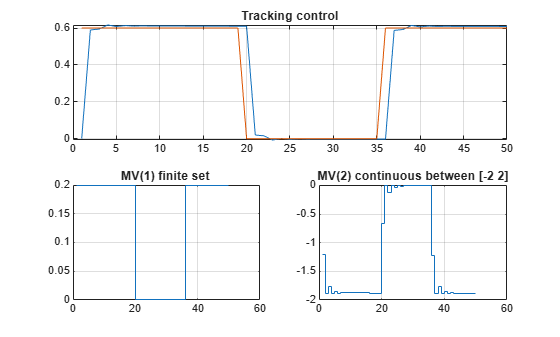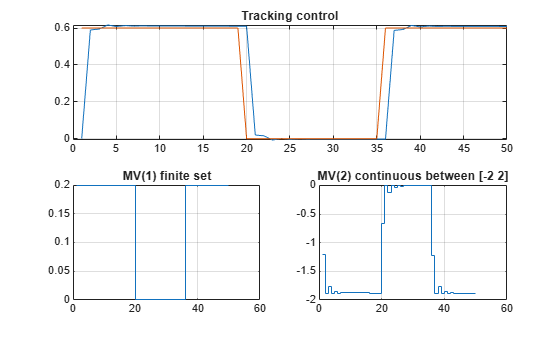# Solve a Finite Set MPC Problem in MATLAB

This example shows how to solve, in MATLAB®, an MPC problem in which some manipulated variables belong to a finite (discrete) set.

### Create a Plant Model

Fix the random generator seed for reproducibility.

`rng(0);`

Create a discrete-time strictly proper plant with 4 states, two inputs and one output.

```plant = drss(4,1,2); plant.D = 0;```

Set the sampling time to 0.1s, and increase the control authority of the first input, to better illustrate its control contribution.

```plant.Ts = 0.1; plant.B(:,1)=plant.B(:,1)*2;```

### Design the MPC Controller

Create an MPC controller with one second sampling time, `20` steps prediction horizon and `5` steps control horizon.

`mpcobj = mpc(plant,0.1,20,5);`
```-->"Weights.ManipulatedVariables" is empty. Assuming default 0.00000. -->"Weights.ManipulatedVariablesRate" is empty. Assuming default 0.10000. -->"Weights.OutputVariables" is empty. Assuming default 1.00000. ```

Specify the first manipulated variable as belonging to a set of seven possible values (you could also specify the type as an integer using the instruction `mpcobj.MV(1).Type = 'integer';`)

`mpcobj.MV(1).Type = [-1 -0.7 -0.3 0 0.2 0.5 1];`

Use rate limits to enforce maximum increment and decrement values for the first manipulated variable.

```mpcobj.MV(1).RateMin = -0.5; mpcobj.MV(1).RateMax = 0.5;```

Set limits on the second manipulated variable, whose default type (continuous) has not been changed.

```mpcobj.MV(2).Min = -2; mpcobj.MV(2).Max = 2;```

### Simulate the Closed Loop Using the `sim` Command and Plot Results

Set the number of simulation steps.

`simsteps = 50;`

Create an output reference signal equal to zero from steps `20` to `35` and equal to `0.6` before and after.

```r = ones(simsteps,1)*0.6; r(20:35) = 0;```

Simulate the closed loop using the `sim` command. Return the plant input and output signals.

`[YY,~,UU,~,~,~,status] = sim(mpcobj,simsteps,r);`
```-->Assuming output disturbance added to measured output #1 is integrated white noise. -->"Model.Noise" is empty. Assuming white noise on each measured output. ```

Plot results.

```figure(1) subplot(211) % plant output plot([YY,r]); grid title("Tracking control"); subplot(223) % first plant input stairs(UU(:,1)); grid title("MV(1) finite set ") subplot(224) % second plant input stairs(UU(:,2)); grid title("MV(2) continuous between [-2 2]")```As expected, the first manipulated variable is restricted to the values specified in the finite set (with jumps less than the specified limit), while the second one can vary continuously between `-2` and `2`. The plant output tracks the reference value after a few seconds.

### Simulate the Closed Loop Using the `mpcmove` Command and Plot Results

Get handle to `mpcobj` state and initialize plant state.

```xmpc = mpcstate(mpcobj); x = xmpc.Plant;```

Initialize arrays that store signals.

`YY = []; RR = []; UU = []; XX = [];`

Perform simulation using the `mpcmove` command to calculate the control actions.

```for k = 1:simsteps XX = [XX;x']; % store plant state y = plant.C*x; % calculate plant output YY = [YY;y]; % store plant output RR = [RR;r(k)]; % store reference u = mpcmove(mpcobj,xmpc,y,r(k)); % calculate optimal mpc move UU = [UU;u']; % store plant input x = plant.A*x+plant.B*u; % update plant state % is the last line necessary since x=xmpc.Plant gets updated anyway? end```

Plot results.

```figure(2) subplot(211) % plant output plot([YY,r]); grid title("Tracking control"); subplot(223) % first plant input stairs(UU(:,1)); grid title("MV(1) finite set") subplot(224) % second plant input stairs(UU(:,2)); grid title("MV(2) continuous between [-2 2]")```The simulation results are identical as the ones achieved using the `sim` command.